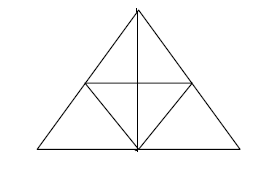### Solved Question Paper for Store Officers in DRDO conducted by UPSC

61.  Q’s mother Y is the sister of P and daughter of M. S is daughter of P and sister of T. How is M related to T?
(a) Father
(b) Mother
(c) Grandfather
(d) Grandfather or Grandmother

62. Find the odd one out from among Oil, Water, Moisture, and Ice?
(a) Water
(b) Oil
(c) Moisture
(d) Ice

63. There are two cylinders. The height of one of them is 32 times the height of the other. If the volume of the shorter cylinder is double the volume of taller cylinder, then what is the ratio of the radii of the shorter cylinder and the taller cylinder?
(a) 6
(b) 4
(c) 8
(d) 16

64. The angle between the hands of a clock at 6:50 a.m. is:
(a) right angle.
(b) straight angle.
(c) obtuse angle.
(d) acute angle.

65. Consider the following figure:What is the number of triangles in the figure given above?
(a) 9
(b) 11
(c) 13
(d) 15

66. A wire of length 12 cm is used to form a triangle of sides 3 cm, 4 cm and 5 cm. The same wire can be used to form a square or a circle. If x, y and z cm2 are areas of the triangle, the square and the circle respectively, then which one of the following is correct?
(a) x > y > z
(b) x < y < z
(c) x < z < y
(d) y < x < z

67. Which one of the following words can NOT be formed by using the letters of the word USEFULNESS?
(a) FUSSES
(b) ELSE
(c) FUNNELS
(d) USES

68. If x, y and z are positive integers such that xyz = 90, then which one of the following is FALSE?
(a) x + y + z = 14
(b) x + y + z = 15
(c) x + y + z = 16
(d) x + y + z = 20

69. One litre of red paint costs Rs. 200 and one litre of green paint costs Rs. 280. A new paint is produced by mixing 5 litre of red paint and 3 litre of green paint. If the cost of mixing the paints is negligible, then what is the cost of one litre of the new paint?
(a) Rs. 210
(b) Rs. 220
(c) Rs. 230
(d) Rs. 240

70. A cylindrical container of capacity 90 litre has two holes on its side wall. One hole is in the middle of its height which drains out 10 litre of water per hour. The other hole is just above the bottom and it drains out 5 litre of water per hour. If the container is filled full with water, then in how much time will it become empty?
(a) 6 hours
(b) 9 hours
(c) 10 hours
(d) 12 hours

Share:

## Featured Post

### UPSC Civil Service Preliminary Paper-1 Previous Year Solved Question Papers

Civil Service Preliminary Paper-1 Previous Year Solved Questions for the year 2019 Civil Service Preliminary Paper-1 Previous Year Solved Qu...Name

Email *

Message *